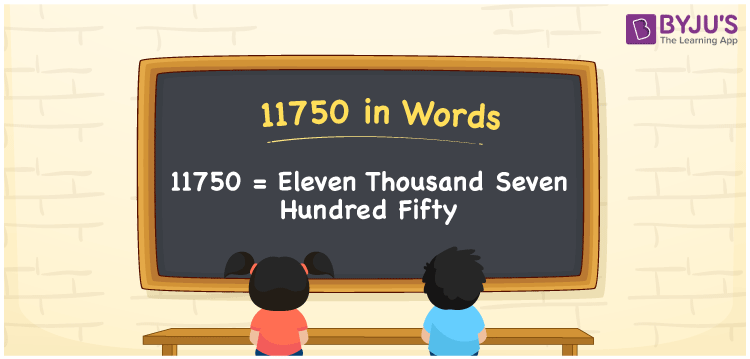# 11750 in Words

11750 in words is Eleven Thousand Seven Hundred Fifty. For example, if you got Rs. 11750 as your prize money, you can write, “I have got Rs. Eleven Thousand Seven Hundred Fifty as my prize money”. In general, the number name of any number can be written using the ones, tens, hundreds, and thousands place of a number. Thus, the place value chart is useful for writing the number 11750 in words.

 11750 in words Eleven Thousand Seven Hundred Fifty Eleven Thousand Seven Hundred Fifty in Numerical Form 11750

## 11750 in English Words## How to Write 11750 in Words?

We can convert 11750 to words using a place value chart. This can be done as follows. The number 11750 has 5 digits, so let’s make a chart that shows the place value up to 5 digits.

 Ten thousands Thousands Hundreds Tens Ones 1 1 7 5 0

Thus, we can write the expanded form as:

1 × Ten thousand + 1 × Thousand + 7 × Hundred + 5 × Ten + 0 × One

= 1 × 10000 + 1 × 1000 + 7 × 100 + 5 × 10 + 0 × 1

= 11750

= Eleven Thousand Seven Hundred Fifty

11750 is the natural number that is succeeded by 11749 and preceded by 11751.

11750 in words – Eleven Thousand Seven Hundred Fifty

Is 11750 an odd number? – No.

Is 11750 an even number? – Yes

Is 11750 a perfect square number? – No

Is 11750 a perfect cube number? – No

Is 11750 a prime number? – No

Is 11750 a composite number? – Yes

## Frequently Asked Questions on 11750 in Words

Q1

### How to write 11750 in words?

11750 in English is written as “Eleven Thousand Seven Hundred Fifty”.
Q2

### Is the number 11750 an odd number?

No, the number 11750 is not an odd number.
Q3

### Is 11750 a composite number?

Yes, 11750 is a composite number.
Test your Knowledge on 11750 in Words